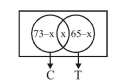# A survey shows that 73% of the persons working in an office like coffee,

Question:

A survey shows that $73 \%$ of the persons working in an office like coffee, whereas $65 \%$ like tea. If $x$ denotes the percentage of them, who like both coffee and tea, then $x$ cannot be:

1. 63

2. 38

3. 54

4. 36

Correct Option: , 4

Solution:

$\mathrm{C} \rightarrow$ person like coffee

$\mathrm{T} \rightarrow$ person like Tea

$\mathrm{n}(\mathrm{C})=73$

$\mathrm{n}(\mathrm{T})=65$

$n(C \cup T) \leq 100$

$n(C)+n(T)-n(C \cap T) \leq 100$$73+65-x \leq 100$

$x \geq 38$

$73-x \geq 0 \Rightarrow x \leq 73$

$65-x \geq 0 \Rightarrow x \leq 65$

$38 \leq \mathrm{x} \leq 65$